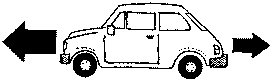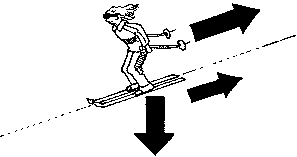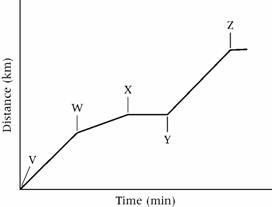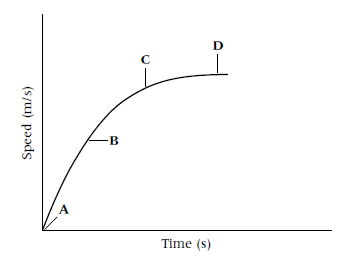9K Quick Quiz

9Ka

1       The speed of a car is a measure of how:

A       far it is travelling.

B       fast it is travelling.

C       long it is travelling.

D       high it is travelling.

2       A bus travelled a distance of 20 km in 2 hours. Its speed was:

A       0.1 km/h.                        B       40 km/h.

C       20 km/h.                         D      10 km/h.

3       The mean speed of something is:

A       its fastest speed.

B       the total distance it travels divided by the time taken.

C       its slowest speed.

D       its speed limit.

4       If a car travels at 50 mph, how long will it take to travel 75 miles?

A       1.5 hours                        B       0.5 hours

C       0.67 hours                      D      67 minutes

9Kb

1       Balanced forces:

A       make objects speed up.

B       make objects slow down.

C       do not change the speed of moving objects.

D       only affect stationary objects.

2       This drawing shows two forces on a car. What will happen to the speed of the car?A       It will accelerate.

B       It will slow down.

C       It will decelerate.

D       It will stay the same.

3       What are forces X and Y?Y X

A       X is air resistance, Y is gravity.

B       X is air resistance, Y is friction.

C       X is gravity, Y is air resistance.

D       X is friction, Y is air resistance.

4       An object will accelerate quickly if it has:

A       a large force and a small mass.

B       a large force and a large mass.

C       a small force and a large mass.

D       a small force and a small mass.

9Kc

1       The air resistance of a car can be reduced by:

A       making the car bigger.

B       making the car heavier.

C       giving it a streamlined shape.

D       putting a roof rack on it.

2       Which statement about air resistance is true?

A       If speed increases, air resistance decreases.

B       If speed increases, air resistance increases.

C       If speed decreases, air resistance increases.

D       Speed has no effect on air resistance.

3       A car needs energy to keep moving because:

A       everything needs a force to keep moving.

B       gravity is trying to slow it down.

C       it needs a force to balance friction and air resistance.

D       weight balances friction.

4       Air resistance on a moving object is caused by:

A       air being attracted to the object.

B       air particles moving around the object.

C       the wind.

D       air particles hitting the object.

9Kd

1       There are changing forces on a skydiver during a jump. Which of these statements is not true?

A       Her weight stays the same.

B       Her air resistance stays the same.

C       Her air resistance is very small at the beginning of the jump.

D       Her air resistance increases as her speed increases.

2       A skydiver reaches a maximum speed when:

A       his air resistance has increased to balance his weight.

B       his air resistance has decreased to balance his weight.

C       his weight has increased to balance the air resistance.

D       his parachute opens.

3       This distance–time graph shows Mrs Martin driving her children to the cinema. Which part of the graph shows where she stopped at some traffic lights?A       V to W

B       W to X

C       X to Y

D       Y to Z

4       This speed–time graph shows the speed of a skydiver. Which letter shows part of the graph where the forces on him are balanced?